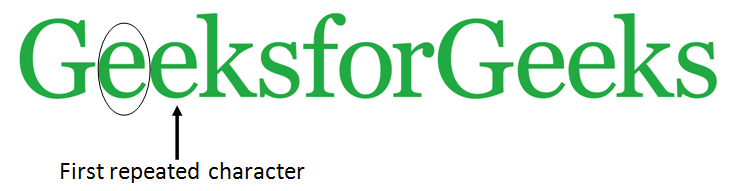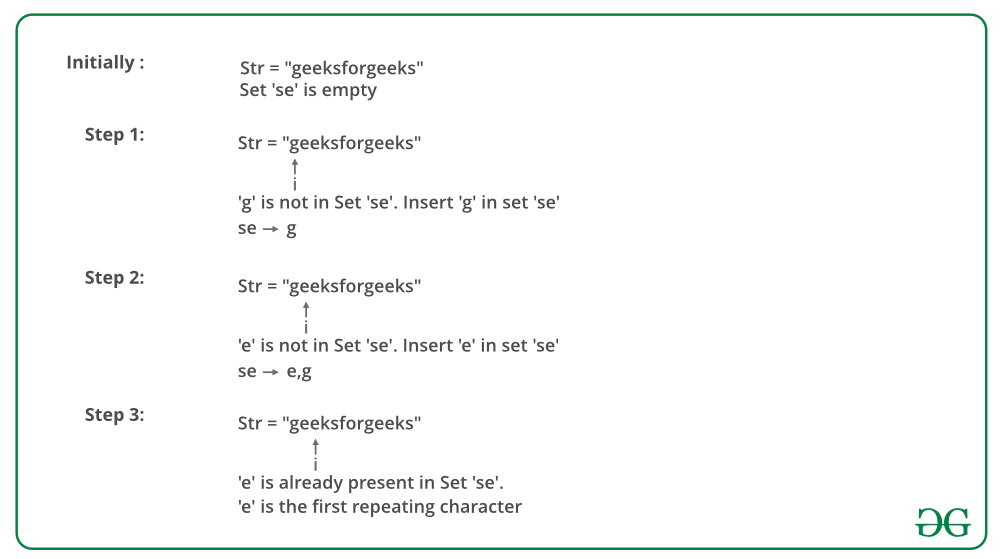# Find the first repeated character in a string

Given a string, find the first repeated character in it. We need to find the character that occurs more than once and whose index of second occurrence is smallest. A variation of this question is discussed here.Examples:

Input: ch = “geeksforgeeks”
Output: e
e is the first element that repeats

Input: str = “hello geeks”
Output: l
l is the first element that repeats

## Recommended: Please solve it on “PRACTICE ” first, before moving on to the solution.

Simple Solution: The solution is to run two nested loops. Start traversing from left side. For every character, check if it repeats or not. If the character repeats, increment count of repeating characters. When the count becomes K, return the character.

Time Complexity of this solution is O(n2)

We can Use Sorting to solve the problem in O(n Log n) time. Following are detailed steps.

• Copy the given array to an auxiliary array temp[].
• Sort the temp array using a O(N log N) time sorting algorithm.
• Scan the input array from left to right. For every element, count its occurrences in temp[] using binary search. As soon as we find a character that occurs more than once, we return the character.

This step can be done in O(N Log N) time.

An efficient solution is to use Hashing to solve this in O(N) time on average.

• Create an empty hash.
• Scan each character of input string and insert values to each keys in the hash.
• When any character appears more than once, hash key value is increment by 1, and return the character.

Below image is a dry run of the above approach:Below is the implementation of the above approach:

## C/C++

 `// CPP program to find the first ` `// repeated character in a string ` `#include ` `using` `namespace` `std; ` ` `  `// Returns first repeating character in str. ` `char` `firstRepeating(string &str) ` `{ ` `    ``// Creates an empty hashset ` `    ``unordered_set<``char``> h; ` ` `  `    ``// Traverse the input array from left to right ` `    ``for` `(``int` `i=0; i

## Java

 `// Java program to find the first ` `// repeated character in a string ` `import` `java.util.*; ` ` `  `class` `Main ` `{ ` `    ``// This function prints the first repeated ` `    ``// character in str[] ` `    ``static` `char` `firstRepeating(``char` `str[]) ` `    ``{ ` `        ``// Creates an empty hashset ` `        ``HashSet h = ``new` `HashSet<>(); ` ` `  `        ``// Traverse the input array from left to right ` `        ``for` `(``int` `i=``0``; i<=str.length-``1``; i++) ` `        ``{ ` `            ``char` `c = str[i]; ` ` `  `            ``// If element is already in hash set, update x ` `            ``// and then break ` `            ``if` `(h.contains(c)) ` `                ``return` `c; ` ` `  `            ``else` `// Else add element to hash set ` `                ``h.add(c); ` `        ``} ` ` `  `        ``return` `'\0'``; ` `    ``} ` ` `  `    ``// Driver method to test above method ` `    ``public` `static` `void` `main (String[] args) ` `    ``{ ` `        ``String str = ``"geeksforgeeks"``; ` `        ``char``[] arr = str.toCharArray(); ` `        ``System.out.println(firstRepeating(arr)); ` `    ``} ` `} `

## Python

 `# Python program to find the first ` `# repeated character in a string ` `def` `firstRepeatedChar(``str``): ` ` `  `    ``h ``=` `{}  ``# Create empty hash ` ` `  `    ``# Traverse each characters in string ` `    ``# in lower case order ` `    ``for` `ch ``in` `str``: ` ` `  `        ``# If character is already present ` `        ``# in hash, return char ` `        ``if` `ch ``in` `h: ` `            ``return` `ch; ` ` `  `        ``# Add ch to hash ` `        ``else``: ` `            ``h[ch] ``=` `0` ` `  `    ``return` `'\0'` ` `  ` `  `# Driver code ` `print``(firstRepeatedChar(``"geeksforgeeks"``)) `

## C#

 `// C# program to find the first  ` `// repeated character in a string  ` `using` `System; ` `using` `System.Collections.Generic; ` ` `  `class` `GFG ` `{ ` `// This function prints the first  ` `// repeated character in str[]  ` `public` `static` `char` `firstRepeating(``char``[] str) ` `{ ` `    ``// Creates an empty hashset  ` `    ``HashSet<``char``> h = ``new` `HashSet<``char``>(); ` ` `  `    ``// Traverse the input array  ` `    ``// from left to right  ` `    ``for` `(``int` `i = 0; i <= str.Length - 1; i++) ` `    ``{ ` `        ``char` `c = str[i]; ` ` `  `        ``// If element is already in hash set,  ` `        ``// update x and then break  ` `        ``if` `(h.Contains(c)) ` `        ``{ ` `            ``return` `c; ` `        ``} ` ` `  `        ``else` `// Else add element to hash set ` `        ``{ ` `            ``h.Add(c); ` `        ``} ` `    ``} ` ` `  `    ``return` `'\0'``; ` `} ` ` `  `// Driver Code ` `public` `static` `void` `Main(``string``[] args) ` `{ ` `    ``string` `str = ``"geeksforgeeks"``; ` `    ``char``[] arr = str.ToCharArray(); ` `    ``Console.WriteLine(firstRepeating(arr)); ` `} ` `} ` ` `  `// This code is contributed by Shrikant13 `

## PHP

 ` `

Output:

```e
```

Similar Problem: finding first non-repeated character in a string.

This article is contributed by Afzal Ansari. If you like GeeksforGeeks and would like to contribute, you can also write an article using contribute.geeksforgeeks.org or mail your article to contribute@geeksforgeeks.org. See your article appearing on the GeeksforGeeks main page and help other Geeks.

Attention reader! Don’t stop learning now. Get hold of all the important DSA concepts with the DSA Self Paced Course at a student-friendly price and become industry ready.

My Personal Notes arrow_drop_up

Improved By : shrikanth13, chitranayal

Article Tags :
Practice Tags :

7

Please write to us at contribute@geeksforgeeks.org to report any issue with the above content.